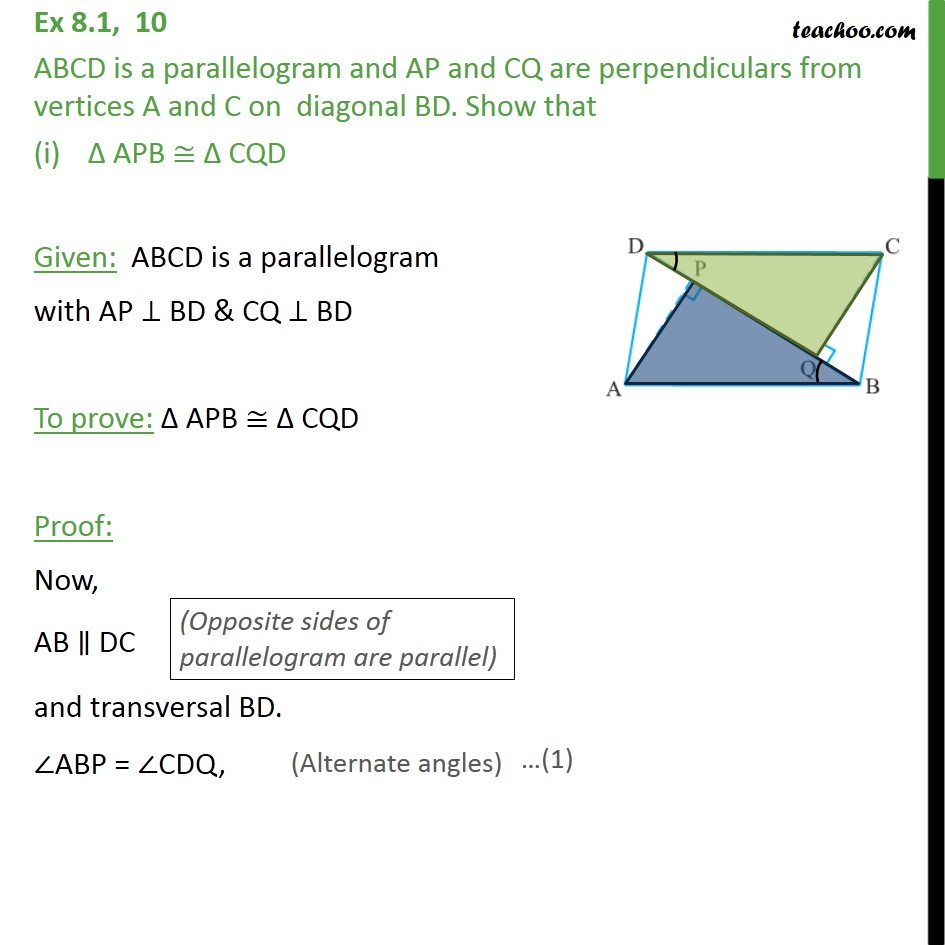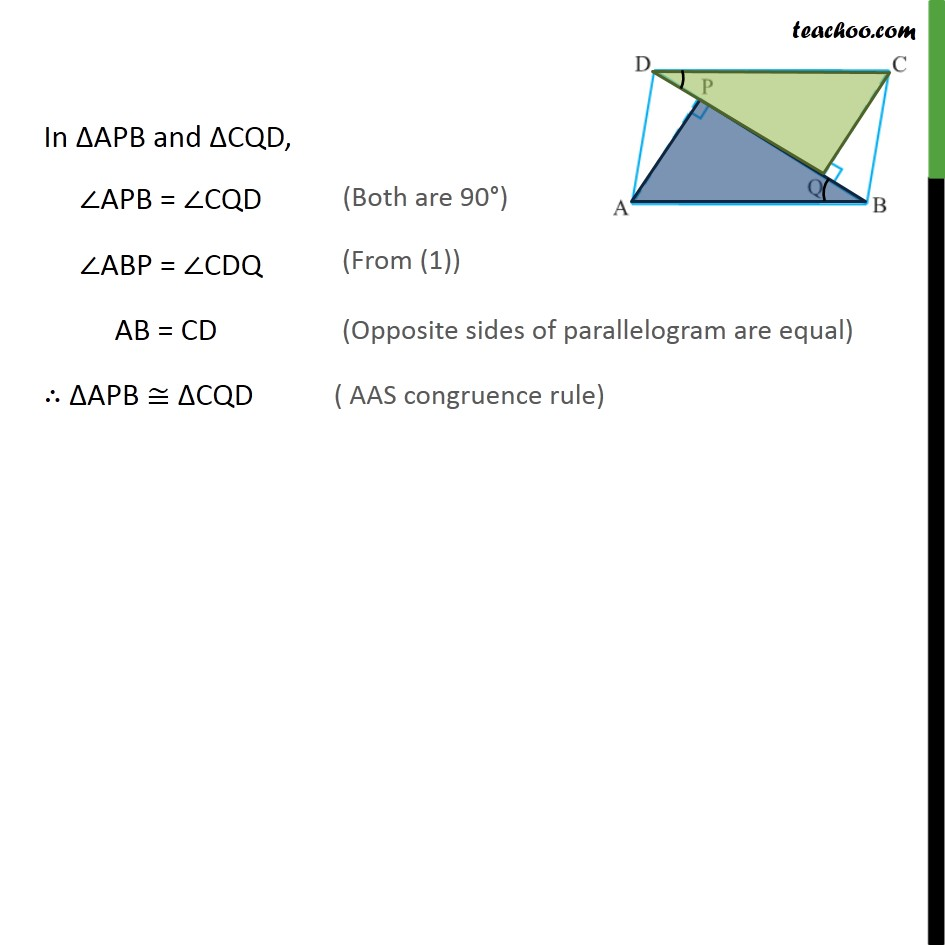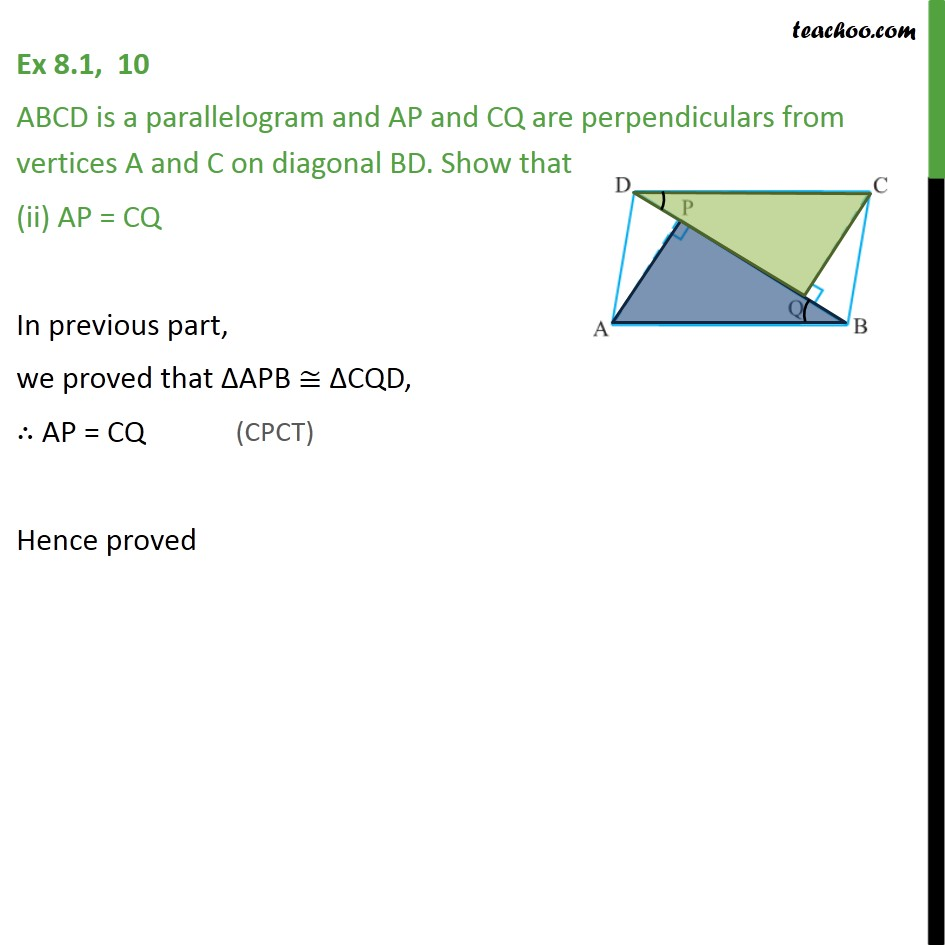1. Chapter 8 Class 9 Quadrilaterals (Term 2)
2. Serial order wise
3. Ex 8.1

Transcript

Ex 8.1, 10 ABCD is a parallelogram and AP and CQ are perpendiculars from vertices A and C on diagonal BD. Show that Δ APB ≅ Δ CQD Given: ABCD is a parallelogram with AP ⊥ BD & CQ ⊥ BD To prove: Δ APB ≅ Δ CQD Proof: Now, AB ∥ DC and transversal BD. ∠ABP = ∠CDQ, In ΔAPB and ΔCQD, ∠APB = ∠CQD ∠ABP = ∠CDQ AB = CD ∴ ΔAPB ≅ ΔCQD Ex 8.1, 10 ABCD is a parallelogram and AP and CQ are perpendiculars from vertices A and C on diagonal BD. Show that (ii) AP = CQ In previous part, we proved that ΔAPB ≅ ΔCQD, ∴ AP = CQ Hence proved